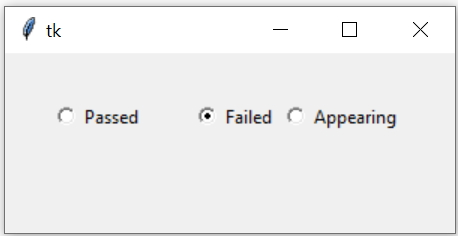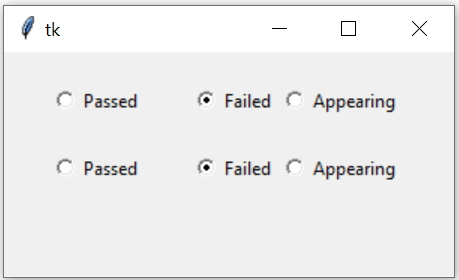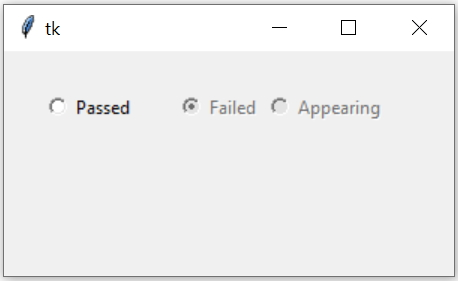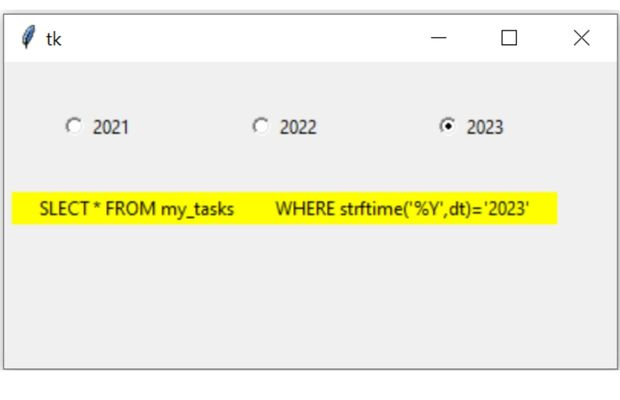Radio buttons are used when options are mutually exclusive and user can't select more then one chice.``````import tkinter as tk
my_w = tk.Tk()
my_w.geometry("300x120")  # Size of the window

r1_v = tk.IntVar()

r1 = tk.Radiobutton(my_w, text='Passed', variable=r1_v, value=1)

r2 = tk.Radiobutton(my_w, text='Failed', variable=r1_v, value=0)
r2.grid(row=1,column=2)

r3 = tk.Radiobutton(my_w, text='Appearing', variable=r1_v, value=5)
r3.grid(row=1,column=3)

my_w.mainloop()  # Keep the window open``````
Managing Radio buttons in Tkinter GUI by setting and reading selected values with options to disable

In above code we have linked the variable IntVar() r1_v to the radiobutton. This variable is common to all three radio buttons. ( In case of Checkbuttons the associated variables are different).

The value option is different for all the three radio buttons. This value will be associated to variable r1_v once the radio button is selected.

At any point of time maximum one radio button can be selected. ( We can select more than one Checkbuttons )

## Collecting and Setting the values of Radiobutton

How to read the status ( value of the associated variable ) of the radio button.
``my_val=r1_v1.get() #r1_v1 is the variable connected to radiobutton ``
How to set the value of the radiobutton ( to select or Unselect )?
``r1_v1.set(1)``
We will use the above concepts. Note that radiobutton can have values 1 or 0 or any other integer( integer variable ), Yes or No or any other string ( string variable) , True or False ( Boolean variable ). We will learn all three types here.

Capturing click event of checkbox and display the value of the variable
``````import tkinter as tk
my_w = tk.Tk()
my_w.geometry("500x500")  # Size of the window

def my_upd():

r1_v = tk.IntVar() # We used integer variable here

r1 = tk.Radiobutton(my_w, text='Passed', variable=r1_v, value=1,command=my_upd)
r1.grid(row=1,column=1)

r2 = tk.Radiobutton(my_w, text='Failed', variable=r1_v, value=0,command=my_upd)
r2.grid(row=1,column=2)

r3 = tk.Radiobutton(my_w, text='Appearing', variable=r1_v, value=5,command=my_upd )
r3.grid(row=1,column=3)

my_w.mainloop()  # Keep the window open``````
Capturing click event of Radiobutton and display the string variable
``````import tkinter as tk
my_w = tk.Tk()
my_w.geometry("500x500")  # Size of the window

def my_upd():

r1_v = tk.StringVar()  # We used string variable here
r1_v.set('Passed')     # Can assign value Appear or Failed

r1 = tk.Radiobutton(my_w, text='Passed', variable=r1_v, value='Passed',command=my_upd)
r1.grid(row=1,column=1)

r2 = tk.Radiobutton(my_w, text='Failed', variable=r1_v, value='Failed',command=my_upd)
r2.grid(row=1,column=2)

r3 = tk.Radiobutton(my_w, text='Appearing', variable=r1_v, value='Appear',command=my_upd )
r3.grid(row=1,column=3)

my_w.mainloop()  # Keep the window open``````
Now let us use one Boolean variable
``````import tkinter as tk
my_w = tk.Tk()
my_w.geometry("500x500")  # Size of the window

def my_upd():

r1_v = tk.BooleanVar()   # We assigned Boolean variable here
r1_v.set(True) # Can assign False

r1 = tk.Radiobutton(my_w, text='Passed', variable=r1_v, value=True,command=my_upd)
r1.grid(row=1,column=1)

r2 = tk.Radiobutton(my_w, text='Failed', variable=r1_v, value=False,command=my_upd)
r2.grid(row=1,column=2)

my_w.mainloop()  # Keep the window open``````

## How to set a default value ( select or unselect ) to a radiobutton

Check the code written above (for click event of Radiobutton and display the string variable) , we used one line to set the value of variable r1_v like this.
``r1_v.set('Passed') # Can assign value Appear or Failed``
We can change above code to set the radio button to a different selection.

We can remove all user selections by assigning the variable to None. Here we have used r1_v as variable option of the radiobuttons.
``r1_v.set(None) ``

Using all above knowledge let us try to make second group of radio buttons check or uncheck based on updating of first group of radiobuttons. Here we need to read the data of first radiobutton and then copy the same to second radiobutton.``````import tkinter as tk
my_w = tk.Tk()
my_w.geometry("500x500")  # Size of the window

def my_upd():
r2_v.set(r1_v.get())  # read from first group and set the second group

r1_v = tk.StringVar()
r1_v.set('Passed') # (default value ) Can assign value Appear or Failed

r1 = tk.Radiobutton(my_w, text='Passed', variable=r1_v, value='Passed',command=my_upd)
r1.grid(row=1,column=1)

r2 = tk.Radiobutton(my_w, text='Failed', variable=r1_v, value='Failed',command=my_upd)
r2.grid(row=1,column=2)

r3 = tk.Radiobutton(my_w, text='Appearing', variable=r1_v, value='Appear',command=my_upd )
r3.grid(row=1,column=3)

r2_v = tk.StringVar()
r2_v.set('Passed') # default value

r4 = tk.Radiobutton(my_w, text='Passed', variable=r2_v, value='Passed',command=my_upd)
r4.grid(row=2,column=1)

r5 = tk.Radiobutton(my_w, text='Failed', variable=r2_v, value='Failed',command=my_upd)
r5.grid(row=2,column=2)

r6 = tk.Radiobutton(my_w, text='Appearing', variable=r2_v, value='Appear',command=my_upd )
r6.grid(row=2,column=3)

my_w.mainloop()``````

## Enable or disable radio buttonsThe option state can take three values : normal, disabled , active. We can use config to set the option also.
``````import tkinter as tk
my_w = tk.Tk()
my_w.geometry("300x150")  # Size of the window

r1_v = tk.IntVar()

r1 = tk.Radiobutton(my_w, text='Passed', variable=r1_v, value=1)

r2 = tk.Radiobutton(my_w, text='Failed', variable=r1_v, value=0)
r2.grid(row=1,column=2)

value=5, state='disabled')
r3.grid(row=1,column=3)
r2.config(state='disabled') # active , normal , disabled

my_w.mainloop()  # Keep the window open````````````import datetime
today = datetime.datetime.now() # creating date object
year=today.year # Present Year as Integer

year1 = today.year+1 #Next Year
year2= today.year-1  #Previous Year

import tkinter as tk
my_w = tk.Tk()
my_w.geometry("400x200")  # Size of the window

r1_v = tk.IntVar(value=year) # declaring Intvar

r1 = tk.Radiobutton(my_w, text=year2, variable=r1_v, value=year2)

r2 = tk.Radiobutton(my_w, text=year, variable=r1_v, value=year)

r3 = tk.Radiobutton(my_w, text=year1, variable=r1_v, value=year1)

lb=tk.Label(my_w,text='Query',bg='yellow',width=50)

def my_upd(*args): # on click of radio buttons
WHERE strftime('%Y',dt)='"+str(r1_v.get())+"'"
print(query)
lb.config(text=query)

r1_v.trace('w',my_upd)
my_w.mainloop()``````
Tkinter Radiobuttons to select current next and previous years and to generate SQL query

2. Create one columns of radio buttons representing languages and second column showing same languages with checkboxes. By Selecting the radio button the matching checkbox should select ( check ) , your change in selection of radio buttons should deselect previous one and check the new one

Example : If you select PHP Radio button then PHP checkbox should select. If you change your selection to Python then Python checkbox should select and at the same time PHP should deselect.

Subscribe to our YouTube Channel here

## Subscribe

* indicates required
Subscribe to plus2netplus2net.com